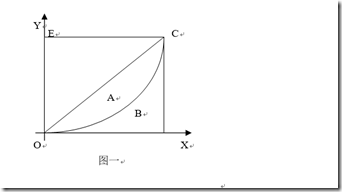# 【推荐算法工程师技术栈系列】推荐系统--数据效果与评估

## 推荐系统上线的基本条件

（1）首先，需要通过离线实验证明它在很多离线指标上优于现有的算法；
（2）然后，需要通过用户调查(或内部人工评测)确定它的用户满意度不低于现有的算法；
（3）最后，通过在线等AB测试确定它在我们关心的指标上优于现有的算法。

## AB实验

（1）AB test 的好处是显而易见的，可以公平获得不同算法实际在线时的性能指标；
（2）AB test 和用户调查一样，同样需要考虑到分布的随机，尽量要将与最终指标有相关性的因素都列出来，总而言之就是切分流量是AB test 的关键；
（3）AB test 的一个重要缺点就是实验周期长，这样才能得到可靠的结果，因此AB test 不应该测试所有的算法，而是只测试在离线实验和用户调查中表现很好的算法；
（4）如果有用户标签库的话，会极大的帮助在线实验。

## 数据指标

### 覆盖率

$Coverage = \frac{|\cup_{u \in U} R(u)|}{|I|} ;其中U是用户集合，I是物品集合，R(u)是为用户u推荐的N个物品的集合$1905年，统计学家洛伦茨提出了洛伦茨曲线，如图一。将社会总人口按收入由低到高的顺序平均分为10个等级组，每个等级组均占10％的人口，再计算每个组的收入占总收入的比重。然后以人口累计百分比为横轴，以收入累计百分比为纵轴，绘出一条反映居民收入分配差距状况的曲线，即为洛伦茨曲线。

$Gini = \frac{1}{n-1} \sum^n_{j=1} (2j-n-1)p(j) ;其中p(j)是从小到大排序的物品列表中第j个物品被推荐的比例，也即p(j)=\frac{物品j被推荐次数}{\sum^n_{j=1}物品j被推荐次数}$

### AUC及gAUC

AUC（Area under curve）是机器学习常用的二分类评测手段，直接含义是ROC曲线下的面积;进一步说其实就是随机抽出一对样本（一个正样本，一个负样本），然后用训练得到的分类器来对这两个样本进行预测，预测得到正样本的概率大于负样本概率的概率。ROC曲线的横轴为假正率（False Positive Rate,FPR)；纵轴为“真正率”(True Positive Rate,TPR)

$TPR = \frac{fp}{p} ; FPR = \frac{tp}{n} ; fp是实际为负预测为正的样本个数;tp是实际为正预测也为正的个数;n是真实负样本总数;p是真实正样本总数$

$AUC = \frac{ \sum_{i \in postitiveClass} rank_i - \frac{M(1+M)}{2}}{M+N} ；其中rank_i实代表了样本i预测概率超过的样本的数目(最高的概率的rank为n,第二高的为n-1)；M是正样本个数,N是负样本个数$

AUC反映的是整体样本间的一个排序能力，而实际用户的结果是个性化的，我们更关注的是同一个用户对不同物品间的排序能力,gAUC(group auc)实际是计算每个用户的auc，然后加权平均，最后得到group auc，这样就能减少不同用户间的排序结果不太好比较这一影响。

$gAUC = \frac{\sum_{(u,p)} w_{(u,p)} * AUC_{(u,p)}}{\sum_{(u,p)} w_{(u,p)}}$

## 附录

posted @ 2019-10-13 11:57  混沌战神阿瑞斯  阅读(691)  评论(0编辑  收藏